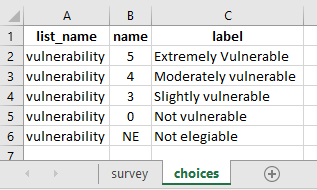# How to calculate the income per preson and prefill a select_one question?

I have a question for income calculation for example I have total income of 500\$ to be div on the HH family size in which I could know the total income for person.

Average Income per Family Member*

IP = income per person

calculates using two questions IP is Less than or equal to \$21 = 5
22 =< IP => 65 = 4
66=< IP => 100 = 3
101=< IP => 179 = 0
\$180 or higher = Not eligible

Welcome to the community, @Ghassan_wilson! Have you gone through our post discussed previously? It should help you solve your issue:

Thank you Kal_Lam for your feedback, I have already taken a look to your post previously but I couldn’t apply the same calculation to me request, is there another solution please

@Ghassan_wilson, so does your survey project have 3 question:

• [integer question] What is the total income of the household?
• [integer question] How many members are there in your household?
• [Calculate] Divide Q1 by Q2 which calculates the income per person (in a household)
• Classify results based on the classification shared previously:
``````calculates using two questions IP is Less than or equal to \$21 = 5
22 =< IP =< 65 = 4
66=< IP =< 100 = 3
101=< IP =< 179 = 0
\$180 or higher = Not eligible
``````

Yes this is what I would like to apply in my form, appreciate if you could share some excel map for the same:

Average Income per Family Member*

IP = income per person

calculates using two questions IP is Less than or equal to \$21 = 5
22 =< IP => 65 = 4
66=< IP => 100 = 3
101=< IP => 179 = 0
\$180 or higher = Not eligible

https://ee.humanitarianresponse.info/x/X5sqWDs5

Hi @Ghassan_wilson
It would be much easier if you tried the excel and then sent it here for us to review and see if you have a problem. This also allows you to practice it out.

Regards,
Stephane

Dear @stephanealoo

it is not working; I followed the same method that have been advised by @Kal_Lam

livelihood3.xlsx (11.7 KB)

@Ghassan_wilson, maybe you could do it as outlined in the image shared below:

#### In the choices tab of your xlform:#### Data entry screen as seen in Enketo:

Reference xlsform:

Livelihood.xlsx (11.5 KB)